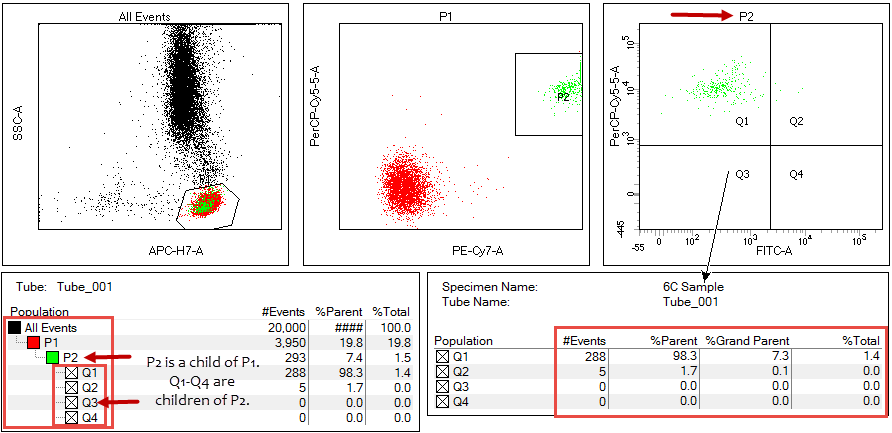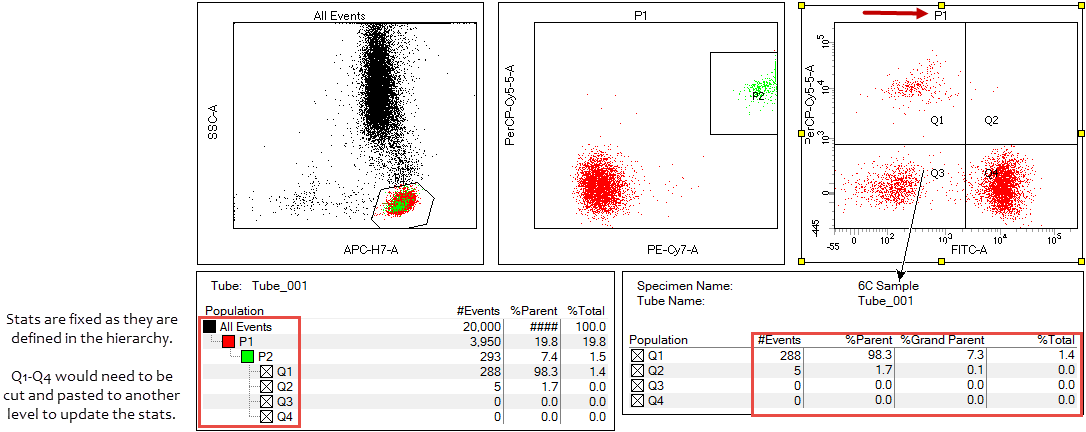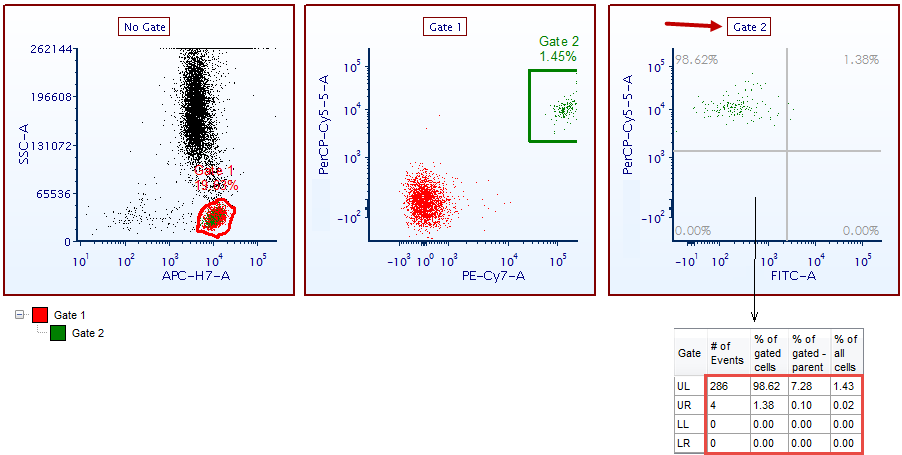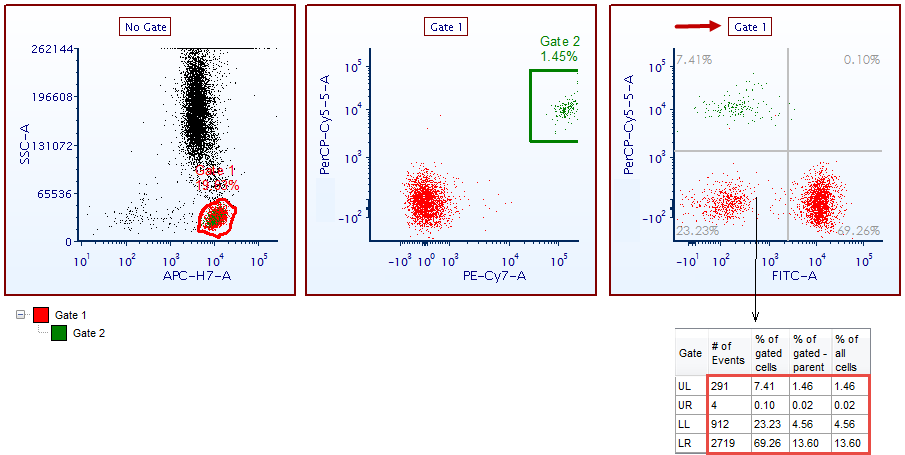# How are statistics in FCS Express calculated compared to how they are calculated in BD FACSDiva?

FCS Express 5 and greater includes the Percent of gate - Parent and Percent of gate - Grandparent Statistics:

Rather than name these statistics plainly Percent Grandparent or Percent Great Grandparent, we need to look into how statistics are calculated in FCS Express 5 and later versus other analysis programs. For comparison, we will use BD FACSDiva (TM).

In BD FACSDiva, statistics are determined by the gates created as they relate to the population hierarchy. Once gates are created as children of other gates, their statistics will be determined by this relationship. It's important to note here that quadrants and markers are created as gates by default in BD FACSDiva.  It is also important to note that BD FACSDiva primarily functions with a single data file at a time, particularly if you are working through a Global Worksheet.

The image below shows a basic analysis of two gates and quadrants. The gate, P1, is the parent of P2, and P2 is the parent of the quadrants, Q1-Q4. As you can see, the statistics are calculated to reflect this hierarchical relationship.However, if the gate applied to the FITC vs PerCP Cy5.5 plot is changed, the statistics stay the same.If this relationship needs to be changed or updated, the gates would need to be rearranged to provide the necessary interpretation of the data being analyzed.

However, that would mean deleting gates and quadrants, or cutting and pasting the gates within the population hierarchy to do this. Because of this static relationship between statistics and the hierarchy, the sources of the statistics % Grandparent and % Great Grandparent will be consistent.

In FCS Express, statistics are updated to reflect the gate applied to a plot.

Below is an image analyzing the same data as above, with a similar gating strategy and hierarchy.When the gate that is applied to the FITC vs PerCP Cy5.5 plot is changed, you can see that the statistics in the quadrant statistics table will update to reflect the application of the new gate.Because of these dynamics, the names of the statistics need to reflect their actual relationship. For FCS Express, the statistic is based on the parent or grandparent of the gate that is applied to the plot for which the statistics are being calculated.

In other words, if Gate 2 is applied to a plot, the statistic for % of gated - parent or % of gated -grandparent will be a statistic that reflects the parent of Gate 2 or the grandparent of Gate 2.

You can find more detail regarding this set of statistics here.